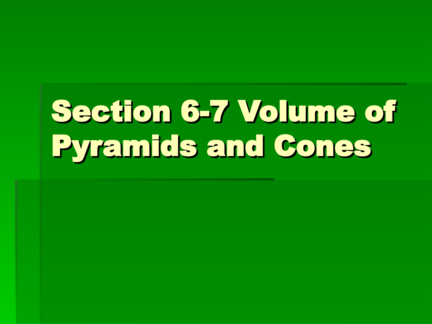# Volume of Pyramids and ConesContributed by:This pdf includes the following topics:-
Volume of pyramids
Rectangular Pyramid
Triangular Pyramid
Cones
1. Section 6-7 Volume of
Pyramids and Cones
2. Rectangular Pyramid
 V=(1/3)lwh
 A rectangular pyramid is the ones that
you would view in Egypt built by
Egyptians.
3. Triangular Pyramid
 V=(1/3)(1/2)lwh
 A triangular pyramid has a base of a
triangle which is what makes it different
from a rectangular pyramid.
4.  V=1/3Bh
 A cone has a circular base. (An ice
cream cone.)
5.  1.) Find the volume of a triangular
pyramid with the height of 4.6cm., width
of 3.9cm. And the length of 1.7cm.
 2.) Find the volume of a rectangular
pyramid with a height of 1.6in., base of
0.8in. And length of 0.4in.
 3.) What does a cone resemble?
6.  http://www.pbs.org/wgbh/nova/pyramid/e
xplore/khufu.html
 http://mathforum.org/dr.math/faq/
formulas/faq.cone.html
 http://www.mathsteacher.com.au/year8/c
h10_geomcons/10_pyramid/spheres.htm